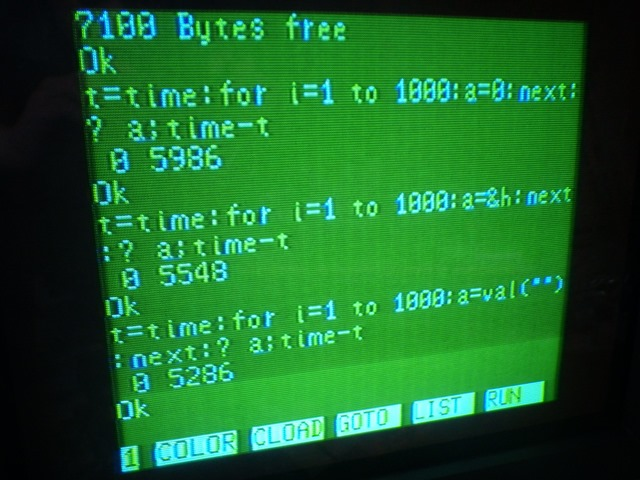# The fastest way to zero in Color BASIC.

In my Optimizing Color BASIC series, one of the things I learned was how much faster it was to use HEX values instead of decimal values. For example:

```A=65535
A=&HFFFF```

Even though the second statement is one character more to parse, it is still much faster since it’s easier for the interpreter to calculate base-16 values than base-10. This was even the case for zero:

```A=0
A=&H0```

Somewhere in the comments, CoCoSDC creator Darren Atkinson let me know about using just a period for zero. I wrote tested that in part 9.

Recently (March 28), Carlos Comacho tagged me in the Facebook CoCo group about something he found on a NEC PC-6001 Z-80 computer testing various ways to set something to zero. Here is the screenshot he shared:

Using a decimal 0 and HEX &H0 were tested, as well as using VAL(“”). Huh?

In the CoCo 3 BASIC quick reference guide, I find this entry:

I am aware of using this to convert a STRING to a number, such as when using LINE INPUT:

```10 LINE INPUT "ENTER YOUR AGE:";A\$
20 A=VAL(A\$)
30 IF A=42 THEN PRINT "ULTIMATE AGE!"```

It also handles decimal values, such as A=VAL(“12.34”). And I guess I knew that if you passed it an empty string, it would return zero because pressing ENTER on a LINE INPUT prompt that then went into VAL would return 0…

## Let’s do nothing!

Of course I had to benchmark this and see what CoCo did with it! Here’s the current version of my BASIC benchmark test:

```0 REM BENCH0.BAS
5 DIM TE,TM,B,A,TT
10 FORA=0TO3:TIMER=0:TM=TIMER
20 FORB=0TO1000
30 Z=0
70 NEXT
80 TE=TIMER-TM:PRINTA,TE
90 TT=TT+TE:NEXT:PRINTTT/A:END```

Running this produces a value of 189. Next we try HEX &H0:

```0 REM BENCHX0.BAS
5 DIM TE,TM,B,A,TT
10 FORA=0TO3:TIMER=0:TM=TIMER
20 FORB=0TO1000
30 Z=&H0
70 NEXT
80 TE=TIMER-TM:PRINTA,TE
90 TT=TT+TE:NEXT:PRINTTT/A:END```

That shows 174. Now let’s do the one with the period:

```0 REM BENCHDOT.BAS
5 DIM TE,TM,B,A,TT
10 FORA=0TO3:TIMER=0:TM=TIMER
20 FORB=0TO1000
30 Z=.
70 NEXT
80 TE=TIMER-TM:PRINTA,TE
90 TT=TT+TE:NEXT:PRINTTT/A:END```

That one gives me 147 – the fastest so far! And lastly, the silly VAL(“”) empty quote thing:

```0 REM BENCHVAL.BAS
5 DIM TE,TM,B,A,TT
10 FORA=0TO3:TIMER=0:TM=TIMER
20 FORB=0TO1000
30 Z=VAL("")
70 NEXT
80 TE=TIMER-TM:PRINTA,TE
90 TT=TT+TE:NEXT:PRINTTT/A:END```

This one gives me 212, even slower than decimal 0.

It looks like, on Color BASIC at least, the VAL(“”) trick is not useful, but I appreciate Carlos letting me know about it.

Have you seen this on any other flavor of BASIC? Let me know in the comments.

Until next time…

This site uses Akismet to reduce spam. Learn how your comment data is processed.